Courses

# Design Of V Belt Drives Mechanical Engineering Notes | EduRev

## Mechanical Engineering : Design Of V Belt Drives Mechanical Engineering Notes | EduRev

The document Design Of V Belt Drives Mechanical Engineering Notes | EduRev is a part of the Mechanical Engineering Course Machine Design.
All you need of Mechanical Engineering at this link: Mechanical Engineering

V - Belt Drives

Among flexible machine elements, perhaps V-belt drives have widest industrial application. These belts have trapezoidal cross section and do not have any joints. Therefore, these belts are manufactured only for certain standard lengths. To accommodate these belts the pulleys have V shaped grooves which makes them relatively costlier. Multiple groove pulleys are available to accommodate number of belts, when large power transmission is required. V-belt drives are most recommended for shorter center distances. In comparison to flat belt drives, these drives are slightly less efficient. V belt can have transmission ratio up to 1:15 and belt slip is very small. As the belts are endless type, V-belt drives do not suffer from any joint failure and are quiet in operation. V-belts constitute fabric and cords of cotton, nylon etc. and impregnated with rubber.

Nomenclature of V-belt

A typical V-belt section is shown in Fig.13.3.1. The geometrical features of the belt section are indicated in the figure. The pitch line, which is also marked as N-A, is the neutral axis of the belt section. The design calculations for Vbelt drives are based on the pitch line or the neutral axis. These belts are available in various sections depending upon power rating.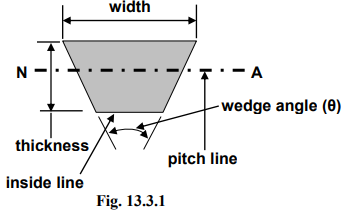Standard V-belt sections

The standard V-belt sections are A, B, C, D and E. The table below contains design parameters for all the sections of V-belt. The kW rating given for a particular section indicates that, belt section selection depends solely on the power transmission required, irrespective of number of belts. If the required power transmission falls in the overlapping zone, then one has to justify the selection from the economic view point also.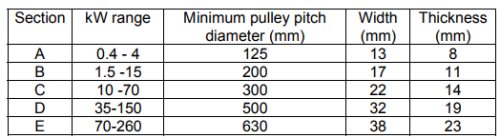As for example, a single belt of B section may be sufficient to transmit the power, instead of two belts of A section. This may increase the cost as well as weight of the pulley, as two- grooved pulley is required. In general, it is better to choose that section for which the required power transmission falls in the lower side of the given range.

Another restriction of choice of belt section arises from the view point of minimum pulley diameter. If a belt of higher thickness (higher section) is used with a relatively smaller pulley, then the bending stress on the belt will increase, thereby shortening the belt life.

Designation of V belt

It has been mentioned that, the calculations for V-belt drives are based on pitch diameter. However, V-belts are designated with nominal inside length (this is easily measurable compared to pitch length). Therefore, to arrive at the inside length, the following relationship is useful.

Inside length + X =Pitch Length       (13.3.1)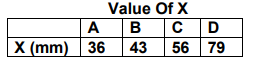For example, a B- section belt with nominal inside length of 1016 mm or 40 inches (nearest value obtained from belt catalogue) is required for a V-belt drive. Then this belt is designated as,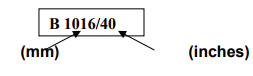V- belt Equation

V-belts have additional friction grip due to the presence of wedge. Therefore, modification is needed in the equation for belt tension. The equation is modified as,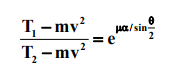(13.3.2)

Where θ is the belt wedge angle

V-belt power rating

Each type of belt section has a power rating. The power rating is given for different pitch diameter of the pulley and different pulley speeds for an angle of wrap of 180ο . A typical nature of the chart is shown below. Here, for example, for pitch diameter of D1 , power rating of the A section belt is kW1, kW2 , kW, kW4 for belt speeds of N1 ,N2 ,N3 ,N4 respectively. Similar tables are available for the belts of other sections.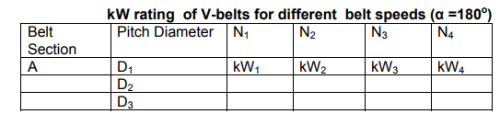V belt design factors

Service Factor

A belt drive is designed based on the design power, which is the modified required power. The modification factor is called the service factor. The service factor depends on hours of running, type of shock load expected and nature of duty.

Hence,

Design Power (P dcs) = service factor (C sev )* Required Power (P)                                                     (13.3.3)

Csev = 1.1 to 1.8 for light to heavy shock.

Modification of kW rating

Power rating of a typical V-belt section requires modification, since, the ratings are given for the conditions other than operating conditions. The factors are as follows,

Equivalent smaller pulley diameter

In a belt drive, both the pulleys are not identical, hence to consider severity of flexing, equivalent smaller pulley diameter is calculated based on speed ratio. The power rating of V-belt is then estimated based on the equivalent smaller pulley diameter (dES ).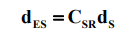(13.3.4)

where, CSR is a factor dependent on the speed ratio.

Angle of wrap correction factor

The power rating of V-belts are based on angle of wrap, α =1800 . Hence, Angle of wrap correction factor ( Cvw ) is incorporated when α is not equal to 180ο .

Belt length correction factor

There is an optimum belt length for which the power rating of a V-belt is given. Let, the belt length be small then, in a given time it is stressed more than that for the optimum belt length. Depending upon the amount of flexing in the belt in a given time a belt length correction factor (CvL) is used in modifying power rating.

Therefore, incorporating the correction factors,

Modified power rating of a belt (kW ) = Power rating of a belt ( kW) x Cvw x Cvl                              (13.3.5)

Selection of V- belt

The transmission ratio of V belt drive is chosen within a range of 1:15

Depending on the power to be transmitted a convenient V-belt section is selected.

The belt speed of a V-belt drive should be around 20m/s to 25 m/s, but should not exceed 30 m/s.

From the speed ratio, and chosen belt speed, pulley diameters are to be selected from the standard sizes available.

Depending on available space the center distance is selected, however, as a guideline,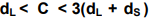(13.3.5)

The belt pitch length can be calculated if C, dL and dS are known. Corresponding inside length then can be obtained from the given belt geometry. Nearest standard length, selected from the design table, is the required belt length.

From section (13.3.7) above, the design power and modified power rating of a belt can be obtained. Therefore,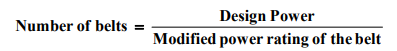(13.3.6)

Sample Problem

Design a flat belt drive for the following data:

Drive: AC motor, operating speed is 1440 rpm and operates for over 10 hours. The equipment driven is a compressor, which runs at 900 rpm and the required power transmission is 20 kW.

Solution

Since it is a V belt drive, let us consider belt speed, v = 25 m/sec.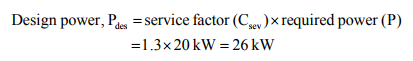The value 1.3 is selected from design data book for the given service condition.

Hence, obvious choice for belt section is C

Now,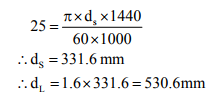standard sizes are,

dS=315 mm and dL=530 mm dS=355 mm and dL = 560 mm.

First combination gives the speed ratio to be 1.68

Second combination gives the speed ratio to be 1.58.

So, it is better to choose the second combination because it is very near to the given speed ratio.

Therefore, selected pulley diameters are dS=355 mm and dL= 560 mm.

Center distance, C should be such that, dL < C < 3(dL + dS )

Let us consider, C = 1500 mm, this value satisfies the above condition. Considering an open belt drive, the belt length,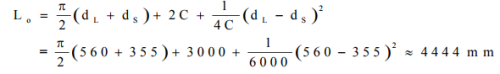Inside length of belt = 4444 – 56 = 4388 mm                            (     from  (13.3.1))

The nearest value of belt length for C-section is 4394 mm (from design data book)

Therefore, the belt designation is C: 4394/173

Power rating (kW) of one C-section belt

Equivalent small pulley diameter is,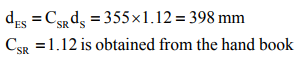For the belt speed of 25 m/sec, the given power rating (kW) = 12.1 kW

For the obtained belt length, the length correction factor Cvl=1.04

D eter m in atio n o f an g le o f w rap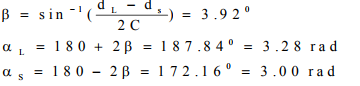For the angle of wrap of 3.00 radian (smaller pulley), the angle of wrap factor, Cvw  is found to 0.98.for a C section belt.

Therefore, incorporating the correction factors,

Modified power rating of a belt (kW )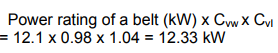Numbe of belts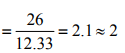2 Numbers of C 4394/173 belts are required for the transmission of 20 kW .

Offer running on EduRev: Apply code STAYHOME200 to get INR 200 off on our premium plan EduRev Infinity!

## Machine Design

57 videos|71 docs|102 tests

,

,

,

,

,

,

,

,

,

,

,

,

,

,

,

,

,

,

,

,

,

;